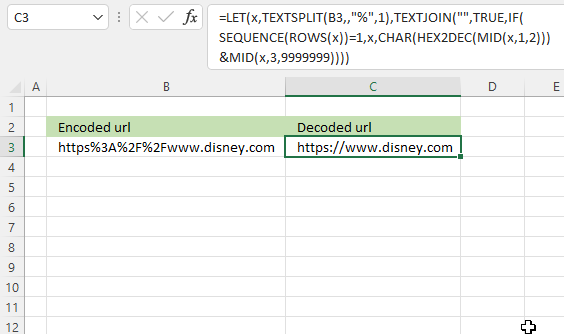Author: Oscar Cronquist Article last updated on October 06, 2022This formula decodes a URL-encoded string, replacing specific percentage symbol (%) and a hexadecimal number with non-alphanumeric characters.

Excel 365 dynamic array formula in cell C3:

=LET(x,TEXTSPLIT(B3,,"%"),TEXTJOIN("",TRUE,IF(SEQUENCE(ROWS(x))=1,x,CHAR(HEX2DEC(MID(x,1,2)))&MID(x,3,99999999999))))

How to use the ENCODEURL function

### Explaining formula

#### Step 1 - Split string into an array based on a given delimiting character (%)

The TEXTSPLIT function splits a string into an array based on delimiting values.

Function syntax: TEXTSPLIT(Input_Text, col_delimiter, [row_delimiter], [Ignore_Empty])

TEXTSPLIT(B3,,"%")

becomes

TEXTSPLIT("https%3A%2F%2Fwww.disney.com",,"%",1)

and returns

{"https";"3A";"2F";"2Fwww.disney.com"}.

#### Step 2 - Count rows in array

The ROWS function calculate the number of rows in a cell range.

Function syntax: ROWS(array)

ROWS(TEXTSPLIT(B3,,"%"))

becomes

ROWS({"https";"3A";"2F";"2Fwww.disney.com"})

and returns

4.

#### Step 3 - Create a sequence from 1 to n

The SEQUENCE function creates a list of sequential numbers.

Function syntax: SEQUENCE(rows, [columns], [start], [step])

SEQUENCE(ROWS(TEXTSPLIT(B3,,"%")))

becomes

SEQUENCE(4)

and returns

{1;2;3;4}.

#### Step 4 - Check if number is equal to 1

SEQUENCE(ROWS(TEXTSPLIT(B3,,"%")))=1

becomes

{1;2;3;4}=1

and returns

{TRUE; FALSE; FALSE; FALSE}.

#### Step 5 - Extract a substring

The MID function returns a substring from a string based on the starting position and the number of characters you want to extract.

Function syntax: MID(text, start_num, num_chars)

MID(TEXTSPLIT(B3,,"%"),1,2)

becomes

MID({"https";"3A";"2F";"2Fwww.disney.com"},1,2)

and returns

{"ht"; "3A"; "2F"; "2F"}.

#### Step 6 - Convert hex value to a number

The HEX2DEC function converts a hexadecimal number to a decimal number.

Function syntax: HEX2DEC(number)

HEX2DEC(MID(TEXTSPLIT(B3,,"%"),1,2))

becomes

HEX2DEC({"ht"; "3A"; "2F"; "2F"})

and returns

{#NUM!; 58; 47; 47}.

#### Step 7 - Convert number to character

The CHAR function converts a number to the corresponding ANSI character determined by your computers character set.

Function syntax: CHAR(text)

CHAR(HEX2DEC(MID(TEXTSPLIT(B3,,"%"),1,2)))

becomes

CHAR({#NUM!; 58; 47; 47})

and returns

{#NUM!; ":"; "/"; "/"}.

#### Step 8 - Extract the last part of each string

The MID function returns a substring from a string based on the starting position and the number of characters you want to extract.

Function syntax: MID(text, start_num, num_chars)

MID(TEXTSPLIT(B3,,"%"),3,9999999))

becomes

MID({"https";"3A";"2F";"2Fwww.disney.com"},3,9999999))

and returns

{"tps";"";"";"www.disney.com"}

#### Step 9 - Concatenate strings

The ampersand character (&) lets you concatenate strings in an Excel formula.

CHAR(HEX2DEC(MID(TEXTSPLIT(B3,,"%"),1,2)))&MID(TEXTSPLIT(B3,,"%"),3,9999999))

becomes

{#NUM!; ":"; "/"; "/"}.&{"tps";"";"";"www.disney.com"}

and returns

{#NUM!;":";"/";"/www.disney.com"}

#### Step 10 - Filter strings based on position

The IF function returns one value if the logical test is TRUE and another value if the logical test is FALSE.

Function syntax: IF(logical_test, [value_if_true], [value_if_false])

IF(SEQUENCE(ROWS(TEXTSPLIT(B3,,"%")))=1,TEXTSPLIT(B3,,"%"),CHAR(HEX2DEC(MID(TEXTSPLIT(B3,,"%"),1,2)))&MID(TEXTSPLIT(B3,,"%"),3,9999999))

becomes

IF({TRUE; FALSE; FALSE; FALSE}, {"https";"3A";"2F";"2Fwww.disney.com"}, {#NUM!;":";"/";"/www.disney.com"})

and returns

{"https"; ":"; "/"; "/www.disney.com"}.

#### Step 11 - Join strings

The TEXTJOIN function combines text strings from multiple cell ranges.

Function syntax: TEXTJOIN(delimiter, ignore_empty, text1, [text2], ...)

TEXTJOIN("",TRUE,IF(SEQUENCE(ROWS(TEXTSPLIT(B3,,"%")))=1,TEXTSPLIT(B3,,"%"),CHAR(HEX2DEC(MID(TEXTSPLIT(B3,,"%"),1,2)))&MID(TEXTSPLIT(B3,,"%"),3,9999999)))

becomes

TEXTJOIN("",TRUE,{"https"; ":"; "/"; "/www.disney.com"})

and returns

"https://www.disney.com".

#### Step 12 - Shorten formula

The LET function lets you name intermediate calculation results which can shorten formulas considerably and improve performance.

Function syntax: LET(name1, name_value1, calculation_or_name2, [name_value2, calculation_or_name3...])

TEXTJOIN("",TRUE,IF(SEQUENCE(ROWS(TEXTSPLIT(B3,,"%")))=1,TEXTSPLIT(B3,,"%"),CHAR(HEX2DEC(MID(TEXTSPLIT(B3,,"%"),1,2)))&MID(TEXTSPLIT(B3,,"%"),3,9999999)))

x - TEXTSPLIT(B3,,"%")

LET(x,TEXTSPLIT(B3,,"%"),TEXTJOIN("",TRUE,IF(SEQUENCE(ROWS(x))=1,x,CHAR(HEX2DEC(MID(x,1,2)))&MID(x,3,99999999999))))

### Get the Excel fileHow-to-decode-url.xlsx